# Dividing PolynomialsPage 2

#### WATCH ALL SLIDES

one less than the degree of the first term of

the dividend. The final value in the row is the

remainder.

Synthetic Division

Slide 8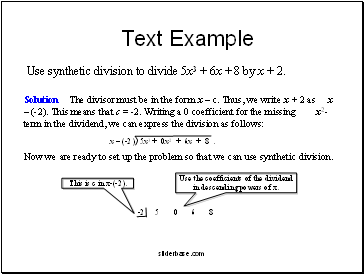## Text Example

Use synthetic division to divide 5x3 + 6x + 8 by x + 2.

Slide 9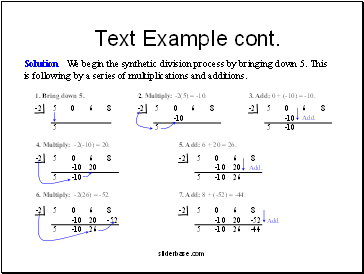Solution We begin the synthetic division process by bringing down 5. This is following by a series of multiplications and additions.

Text Example cont.

Slide 10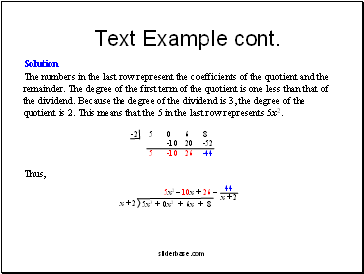Text Example cont.

Slide 11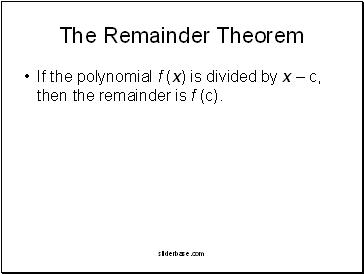## The Remainder Theorem

If the polynomial f (x) is divided by x – c, then the remainder is f (c).

Slide 12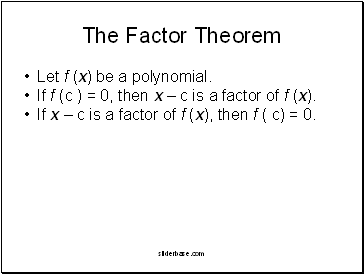## The Factor Theorem

Let f (x) be a polynomial.

If f (c ) = 0, then x – c is a factor of f (x).

If x – c is a factor of f (x), then f ( c) = 0.

Slide 13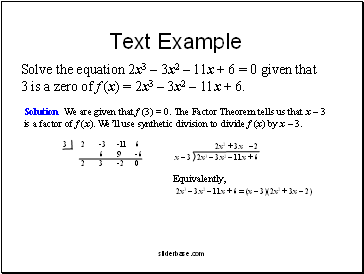Text Example

Solve the equation 2x3 – 3x2 – 11x + 6 = 0 given that 3 is a zero of f (x) = 2x3 – 3x2 – 11x + 6.

Slide 14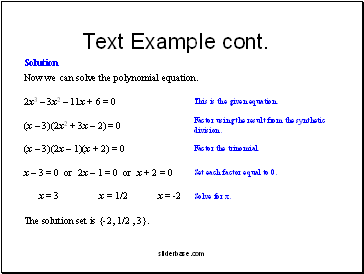Solution

Now we can solve the polynomial equation.

The solution set is {-2, 1/2 , 3}.

Text Example cont.

Go to page:
1  2